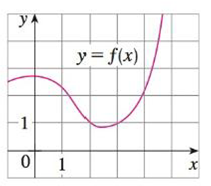Chapter 3.4, Problem 66E

Chapter
Section
Textbook Problem

If f is the function whose graph is shown, let h(x) = f(f(x)) and g(x) = f(x2). Use the graph of f to estimate the value of each derivative.(a) h′(2)(b) g′(2)(a)

To determine

To find: The value h(2).

Explanation

Given:

The function is h(x)=f(f(x)).

Result used: Chain Rule

If h is differentiable at x and g is differentiable at h(x), then the composite function F=gh defined by F(x)=g(h(x)) is differentiable at x and F is given by the product,

F(x)=g(h(x))h(x) (1)

The slope of the line passing through the points (x1,y1) and (x2,y2) is y2y1x2x1.

Calculation:

Obtain the derivative of h(x)=f(f(x)).

h(x)=ddx(h(x))=ddx(f(f(x)))

Apply the chain rule as shown in equation (1),

h(x)=f(f(x))f(x)

Substitute x=2 in the above equation,

h(2)=f(f(2))f(2)

From the given graph it is observed that, f(2)=1.

h(2)=f(1)f(2) (2)

Obtain the values f(1) and f(2)

(b)

To determine

To find: The value g(2).

Still sussing out bartleby?

Check out a sample textbook solution.

See a sample solution

The Solution to Your Study Problems

Bartleby provides explanations to thousands of textbook problems written by our experts, many with advanced degrees!

Get Started

In Exercises 49-62, find the indicated limit, if it exists. 57. limx2x2x6x2+x2

Applied Calculus for the Managerial, Life, and Social Sciences: A Brief Approach

Using the simplex matrix from Problem 2, perform the following. 6. Find the solution.

Mathematical Applications for the Management, Life, and Social Sciences

True or False: f(x)f(a)xa may be interpreted as the instantaneous velocity of a particle at time a.

Study Guide for Stewart's Single Variable Calculus: Early Transcendentals, 8th